### PROC HPGENSELECT Statement

PROC HPGENSELECT <options> ;

The PROC HPGENSELECT statement invokes the procedure. Table 7.1 summarizes the available options in the PROC HPGENSELECT statement by function. The options are then described fully in alphabetical order.

Table 7.1: PROC HPGENSELECT Statement Options

Option

Description

Basic Options

Specifies a global significance level

Specifies the input data set

Limits the length of effect names

Output Options

Displays the Parameter Estimates Correlation Matrix table

Displays the Parameter Estimates Covariance Matrix table

Displays the Iteration History table when no model selection is performed

Displays the summarized Iteration History table when model selection is performed

Displays the summarized Iteration History table when no model selection is performed

Suppresses ODS output

Limits or suppresses the display of classification variable levels

Suppresses computation of the covariance matrix and standard errors

Optimization Options

Tunes the absolute function convergence criterion

Tunes the absolute function difference convergence criterion

Tunes the absolute gradient convergence criterion

Tunes the relative function difference convergence criterion

Tunes the relative gradient convergence criterion

Chooses the maximum number of iterations in any optimization

Specifies the maximum number of function evaluations in any optimization

Specifies the upper limit of CPU time (in seconds) for any optimization

Specifies the minimum number of iterations in any optimization

Specifies whether the objective function is normalized during optimization

Selects the optimization technique

Tolerance Options

Tunes the singularity criterion for Cholesky decompositions

Tunes the singularity criterion for the sweep operator

Tunes the general singularity criterion

User-Defined Format Options

Specifies the file reference for a format stream

You can specify the following options in the PROC HPGENSELECT statement.

ABSCONV=r
ABSTOL=r

specifies an absolute function convergence criterion. For minimization, termination requires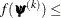r, where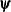is the vector of parameters in the optimization and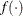is the objective function. The default value of r is the negative square root of the largest double-precision value, which serves only as a protection against overflow.

ABSFCONV=r <n>
ABSFTOL=r <n>

specifies an absolute function difference convergence criterion. For all techniques except NMSIMP, termination requires a small change of the function value in successive iterations: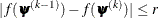Here,denotes the vector of parameters that participate in the optimization, andis the objective function. The same formula is used for the NMSIMP technique, but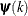is defined as the vertex that has the lowest function value and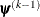is defined as the vertex that has the highest function value in the simplex. The default value is r = 0. The optional integer value n specifies the number of successive iterations for which the criterion must be satisfied before the process can be terminated.

ABSGCONV=r <n>
ABSGTOL=r <n>

specifies an absolute gradient convergence criterion. Termination requires the maximum absolute gradient element to be small: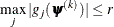Here,denotes the vector of parameters that participate in the optimization, and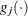is the gradient of the objective function with respect to theth parameter. This criterion is not used by the NMSIMP technique. The default value is r = 1E–8. The optional integer value n specifies the number of successive iterations for which the criterion must be satisfied before the process can be terminated.

ALPHA=number

specifies a global significance level for the construction of confidence intervals. The confidence level is 1 – number. The value of number must be between 0 and 1; the default is 0.05. You can override this global significance level by specifying the ALPHA= option in the MODEL statement or the ALPHA= option in the OUTPUT statement.

CORR

creates the Parameter Estimates Correlation Matrix table. The correlation matrix is computed by normalizing the covariance matrix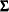. That is, if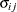is an element of, then the corresponding element of the correlation matrix is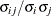, where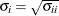.

COV

creates the Parameter Estimates Covariance Matrix table. The covariance matrix is computed as the inverse of the negative of the matrix of second derivatives of the log-likelihood function with respect to the model parameters (the Hessian matrix).

DATA=SAS-data-set

names the input SAS data set for PROC HPGENSELECT to use. The default is the most recently created data set.

If the procedure executes in distributed mode, the input data are distributed to memory on the appliance nodes and analyzed in parallel, unless the data are already distributed in the appliance database. In that case the procedure reads the data alongside the distributed database. For information about the various execution modes, see the section Processing Modes; for information about the alongside-the-database model, see the section Alongside-the-Database Execution.

FCONV=r <n>
FTOL=r <n>

specifies a relative function difference convergence criterion. For all techniques except NMSIMP, termination requires a small relative change of the function value in successive iterations: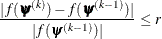Here,denotes the vector of parameters that participate in the optimization, andis the objective function. The same formula is used for the NMSIMP technique, but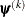is defined as the vertex that has the lowest function value, andis defined as the vertex that has the highest function value in the simplex.

The default value is r =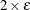, whereis the machine precision. The optional integer value n specifies the number of successive iterations for which the criterion must be satisfied before the process can terminate.

FMTLIBXML=file-ref

specifies the file reference for the XML stream that contains the user-defined format definitions. User-defined formats are handled differently in a distributed computing environment than they are in other SAS products. For information about how to generate an XML stream for your formats, see the section Working with Formats in Chapter 3: Shared Concepts and Topics.

GCONV=r <n>
GTOL=r <n>

specifies a relative gradient convergence criterion. For all techniques except CONGRA and NMSIMP, termination requires that the normalized predicted function reduction be small: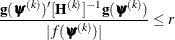Here,denotes the vector of parameters that participate in the optimization,is the objective function, and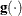is the gradient. For the CONGRA technique (where a reliable Hessian estimate
is not available), the following criterion is used: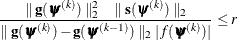This criterion is not used by the NMSIMP technique. The default value is r=1E–8. The optional integer value n specifies the number of successive iterations for which the criterion must be satisfied before the process can terminate.

ITDETAILS

adds to the Iteration History table the current values of the parameter estimates and their gradients. These quantities are reported only for parameters that participate in the optimization. This option is not available when you perform model selection.

ITSELECT

generates the Iteration History table when you perform a model selection.

ITSUMMARY

generates the Iteration History table. This option is not available when you perform model selection.

MAXFUNC=n
MAXFU=n

specifies the maximum number of function calls in the optimization process. The default values are as follows, depending on the optimization technique:

• TRUREG, NRRIDG, NEWRAP: n = 125

• QUANEW, DBLDOG: n = 500

• CONGRA: n = 1,000

• NMSIMP: n = 3,000

The optimization can terminate only after completing a full iteration. Therefore, the number of function calls that are actually performed can exceed n. You can choose the optimization technique by specifying the TECHNIQUE= option.

MAXITER=n
MAXIT=n

specifies the maximum number of iterations in the optimization process. The default values are as follows, depending on the optimization technique:

• TRUREG, NRRIDG, NEWRAP: n = 50

• QUANEW, DBLDOG: n = 200

• CONGRA: n = 400

• NMSIMP: n = 1,000

These default values also apply when n is specified as a missing value. You can choose the optimization technique by specifying the TECHNIQUE= option.

MAXTIME=r

specifies an upper limit of r seconds of CPU time for the optimization process. The default value is the largest floating-point double representation of your computer. The time specified by this option is checked only once at the end of each iteration. Therefore, the actual running time can be longer than r.

MINITER=n
MINIT=n

specifies the minimum number of iterations. The default value is 0. If you request more iterations than are actually needed for convergence to a stationary point, the optimization algorithms might behave strangely. For example, the effect of rounding errors can prevent the algorithm from continuing for the required number of iterations.

NAMELEN=number

specifies the length to which long effect names are shortened. The default and minimum value is 20.

NOCLPRINT<=number>

suppresses the display of the Class Level Information table if you do not specify number. If you specify number, the values of the classification variables are displayed for only those variables whose number of levels is less than number. Specifying a number helps to reduce the size of the Class Level Information table if some classification variables have a large number of levels.

NOPRINT

suppresses the generation of ODS output.

NORMALIZE=YES | NO

specifies whether to normalize the objective function during optimization by the reciprocal of the frequency count of observations that are used in the analysis. This option affects the values that are reported in the Iteration History table. The results that are reported in the Fit Statistics are always displayed for the nonnormalized log-likelihood function. By default, NORMALIZE = NO.

NOSTDERR

suppresses the computation of the covariance matrix and the standard errors of the regression coefficients. When the model contains many variables (thousands), the inversion of the Hessian matrix to derive the covariance matrix and the standard errors of the regression coefficients can be time-consuming.

SINGCHOL=number

tunes the singularity criterion in Cholesky decompositions. The default is 1E4 times the machine epsilon; this product is approximately 1E–12 on most computers.

SINGSWEEP=number

tunes the singularity criterion for sweep operations. The default is 1E4 times the machine epsilon; this product is approximately 1E–12 on most computers.

SINGULAR=number

tunes the general singularity criterion that is applied in sweeps and inversions. The default is 1E4 times the machine epsilon; this product is approximately 1E–12 on most computers.

TECHNIQUE=keyword
TECH=keyword

specifies the optimization technique for obtaining maximum likelihood estimates. You can choose from the following techniques by specifying the appropriate keyword:

CONGRA

DBLDOG

performs a version of double-dogleg optimization.

NEWRAP

performs a Newton-Raphson optimization with line search.

NMSIMP

NONE

performs no optimization.

NRRIDG

performs a Newton-Raphson optimization with ridging.

QUANEW

performs a dual quasi-Newton optimization.

TRUREG

performs a trust-region optimization

The default value is TECHNIQUE=NRRIDG, except for the Tweedie distribution, for which the default value is TECHNIQUE=QUANEW.